# Rate of Change in AP Calculus: Tutoring Solution Chapter Exam

Exam Instructions:

Choose your answers to the questions and click 'Next' to see the next set of questions. You can skip questions if you would like and come back to them later with the yellow "Go To First Skipped Question" button. When you have completed the practice exam, a green submit button will appear. Click it to see your results. Good luck!

### Page 1

#### Question 1 1. The derivative of f(x) is 0 at how many points on the graph below?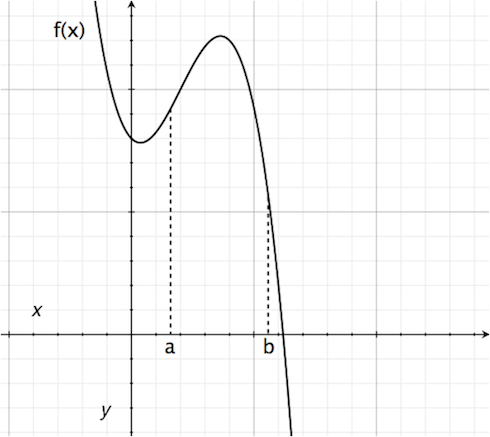#### Question 2 2. Consider the function f(x) given below. According to Rolle's theorem, between x=0 and x=pi, for how many values of x is the instantaneous rate of change equal to 0?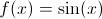#### Question 3 3. What is the rate of change at the point A in the graph below (for y in relation to x)?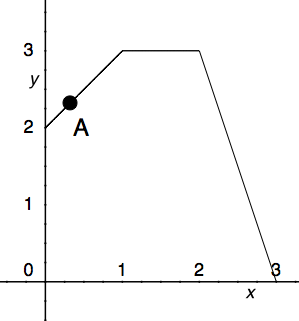#### Question 4 4. Where is f(x) differentiable?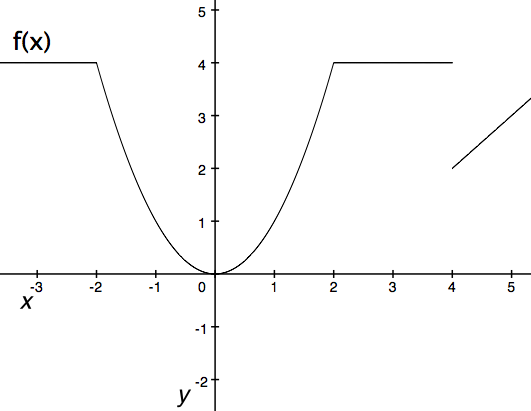#### Question 5 5. What is the rate of change at the point B in the graph below (for y in relation to x)?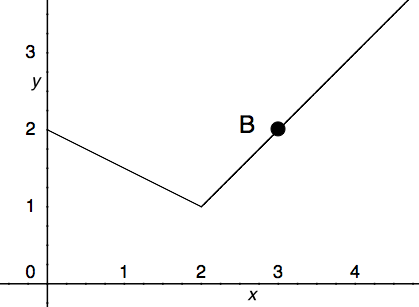### Page 2

#### Question 6 6. Which is greater, the derivative at a or the derivative at b?#### Question 7 7. Which point on the following graph satisfies the mean value theorem between x = 0 and x = 3?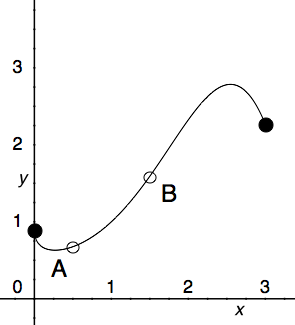#### Question 10 10. What is the rate of change at the point A in the graph below (for y in relation to x)?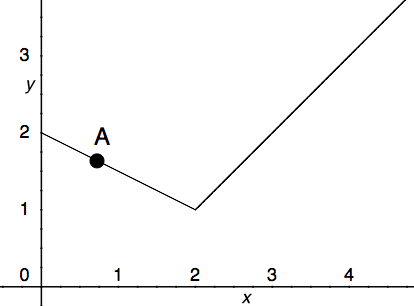### Page 3

#### Question 11 11. What is the derivative of the following?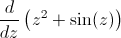#### Question 12 12. Where is f(x) differentiable?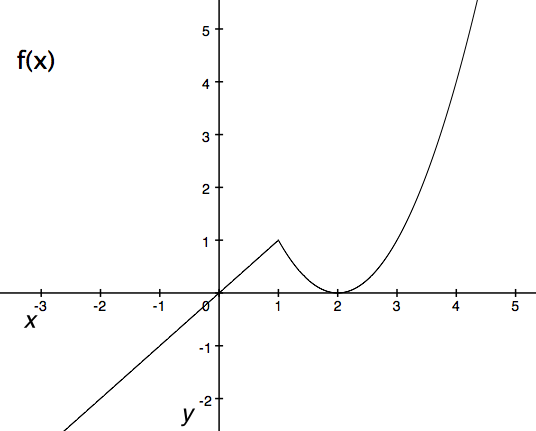#### Question 13 13. According to the mean value theorem, at how many points will the instantaneous rate of change equal the average rate of change between x = 1 and x = 3 on the following graph?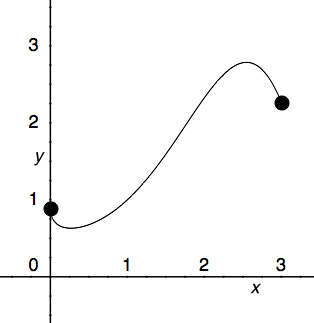#### Question 15 15. Where is y differentiable?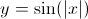### Page 4

#### Question 16 16. What is the value of f(x) at the red circle?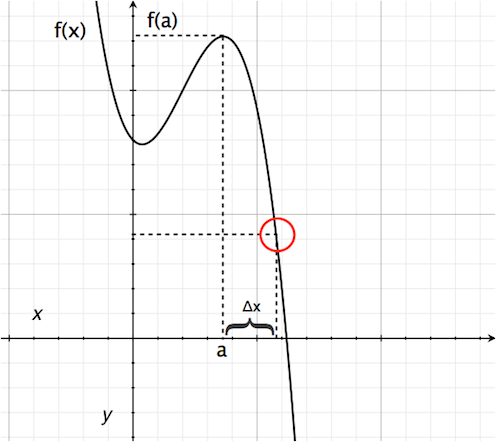#### Question 20 20. What is the rate of change at the point E in the graph below (for y in relation to x)?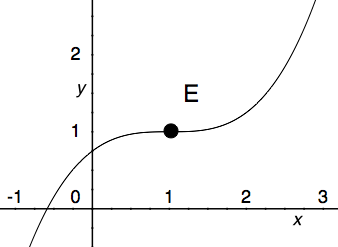### Page 5

#### Question 21 21. Where is f(x) differentiable?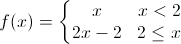#### Question 22 22. What is the derivative of f(t)?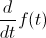#### Question 24 24. At how many points on the graph below is the derivative of f(x)=0?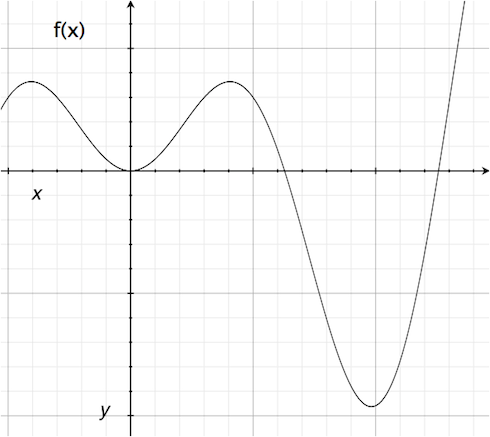#### Question 25 25. What is the rate of change at the point B in the graph below (for y in relation to x)? (In other words, what is the slope of the line from x = 2 to x = 3?)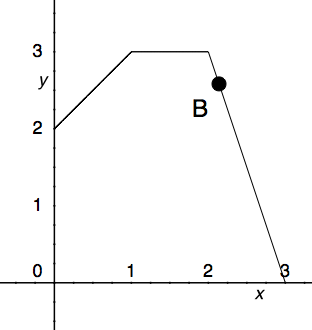### Page 6

#### Question 26 26. Consider the graph and question below..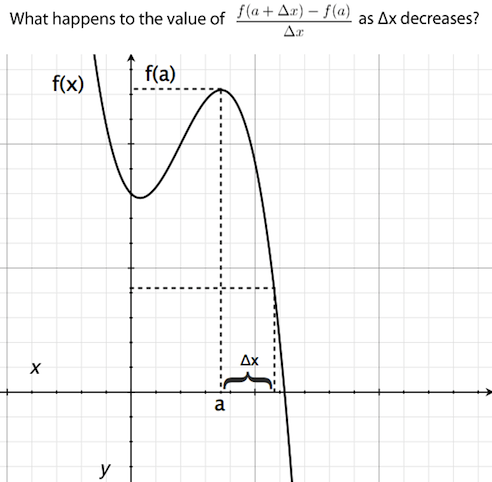#### Question 29 29. Consider the graph below. Between x=C and x=-C, for any value of C, will there be a point where the instantaneous rate of change is 0?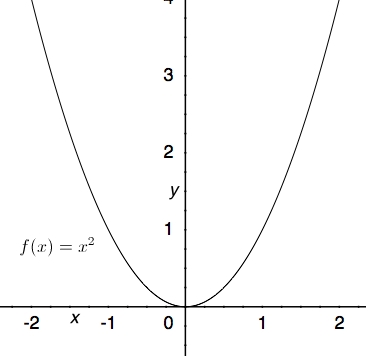#### Question 30 30. What is the approximate instantaneous rate of change at point C?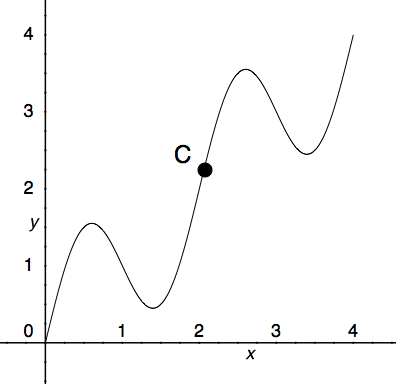#### Rate of Change in AP Calculus: Tutoring Solution Chapter Exam Instructions

Choose your answers to the questions and click 'Next' to see the next set of questions. You can skip questions if you would like and come back to them later with the yellow "Go To First Skipped Question" button. When you have completed the practice exam, a green submit button will appear. Click it to see your results. Good luck!

Support